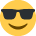# Practice assessment 1 - Question 1 (b)

Hi,

I am having complete mind block on how to work out the total fixed and variable overheads for the below question! Please can someone help and explain?

Question is below:
Units produced: 19,000 - £295,000
Units produced: 23,900 - £324,400

So asks you to calculate the below based on above:
Fixed £ Variable £
21,000
36,000

Please can someone help me on the above?

Thank you• Firstly, we can work out the variable unit cost by using the high-low method. Doing this, we get 4,900 units at £29,400. Divide £29,400 by 4,900 to get the variable unit cost, which would be £6.00.

We can now use this to find the fixed cost. We'll use the 19,000 example. £6.00 * 19,000 is £114,000. Subtract this from £295,000 to find the fixed cost, £181,000. You can replicate this in the 23,900 example too.

So for the calculations for 21,000 and 36,000, the fixed cost would be £181,000, since it doesn't vary based on units produced. Variable costs you just multiply the variable unit cost (£6.00) by the units produced, which equates to £126,000 and £216,000 respectively.

Total costs would, therefore, be £307,000 and £397,000 respectively.

Let me know if something doesn't make sense. Welcome to the AAT forumsMatthew Dearlove MAAT
Currently on CIMA Management Level. Passed P2, F2 and E2. Preparing for November 19 MCS.
• Hello,

Ok so first of all we will have to apply the High-Low costing method, this is because we need to find out variable and fixed costs, we have 2 period figures so we can easily work it out by doing High-Low method;

You need to take the highest units for 1 period – lowest units for 1 period, in this instance is £23,900 - £19,000 = £4,900
Then you look at the total costs, again same principle highest cost – lowest cost; £324,400 - £295,000 = £29,400
Now you simply divide total costs by units; £29,400 / £4,900 = £6.00 – This is variable cost
To check if we are correct;
£6.00 x 23,900 = £143,400. £324,400 - £143,400 = £181,000 Fixed costs
£6.00 x 19,000 = £114,000. £295,000 - £143,400 = £181,000 Fixed Costs
From this we can now see that £6.00 is our variable cost per unit and £181,000 is our fixed costs;

21,000 Units;
£6.00 x 21,000 = £126,000 Variable Cost + £181,000 Fixed Cost = £307,000 Total Cost

36,000 Units;
£6.00 x £36,000 = £216,000 Variable Cost + £181,000 Fixed Cost = £397,000 Total Cost

I hope this helps!
Kind Regards,

Norvydas Valavicius.